28 Tests and Conditionals
 In:=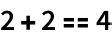Out=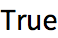In:=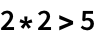Out=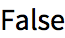The function If lets you choose to give one result if a test is True, and another if its False.
Since the test gives True, the result of the If is x:
 In:=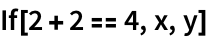Out=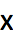By using a pure function with /@, we can apply an If to every element of a list.
If an element is less than 4, make it x, otherwise make it y:
 In:=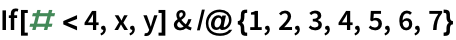Out=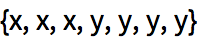You can also test for less than or equal using , which is typed as <=.
If an element is less than or equal to 4, make it x; otherwise, make it y:
 In:=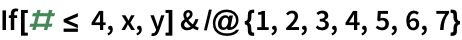Out=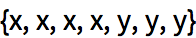This makes an element x only if it is equal to 4:
 In:=Out=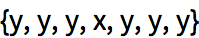If an element is not equal to 4, make it x; otherwise, make it y:
 In:=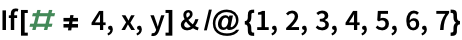Out=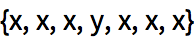Select elements in the list that are greater than 3:
 In:=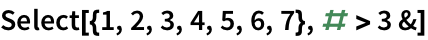Out=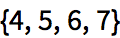Select elements that are between 2 and 5:
 In:=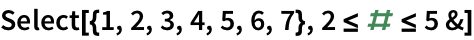Out=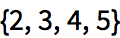Beyond size comparisons like <, > and ==, the Wolfram Language includes many other kinds of tests. Examples are EvenQ and OddQ, which test whether numbers are even or odd. (The Q indicates that the functions are asking a question.)
4 is an even number:
 In:=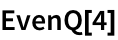Out=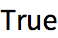Select even numbers from the list:
 In:=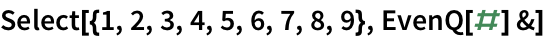Out=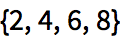In this case, we dont need the explicit pure function:
 In:=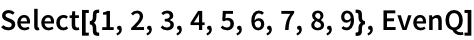Out=IntegerQ tests whether something is an integer; PrimeQ tests whether a number is prime.
Select prime numbers:
 In:=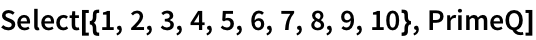Out=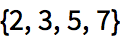Sometimes we need to combine tests. && represents and, || represents or and ! represents not.
Select elements of the list that are both even and greater than 2:
 In:=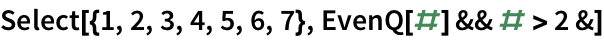Out=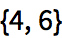Select elements that are either even or greater than 4:
 In:=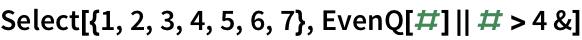Out=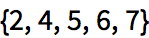Select elements that are not either even or greater than 4:
 In:=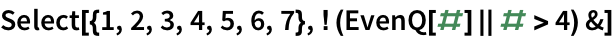Out=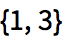The space between letters isnt a letter; nor is !:
 In:=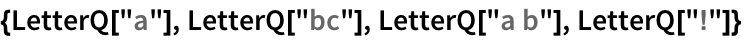Out=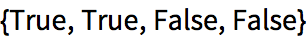In:=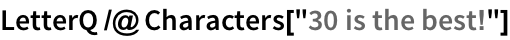Out=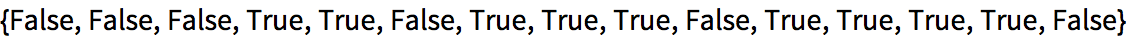Select the characters that are letters:
 In:=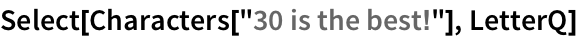Out=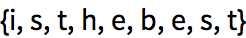In:=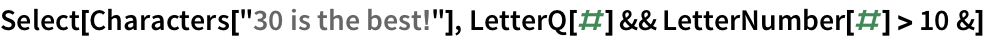Out=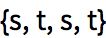You can use Select to find words in English that are palindromes, meaning that they are the same if you reverse them.
 In:=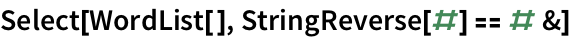Out=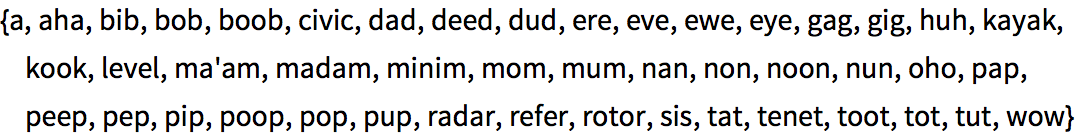MemberQ tests whether something appears as an element, or member, of a list.
5 appears in the list {1, 3, 5, 7}:
 In:=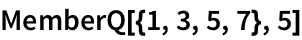Out=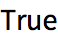Select numbers in the range 1 to 100 whose digit sequences contain 2:
 In:=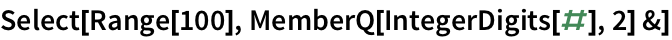Out=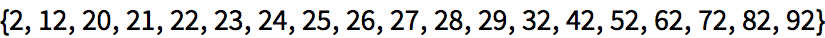ImageInstanceQ is a machine-learning-based function that tests whether an image is an instance of a particular kind of thing, like a cat.
Test if an image is of a cat:
 In:=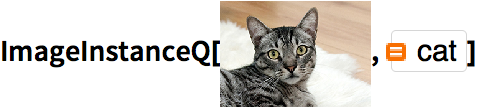Out=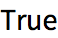Select images of cats:
 In:=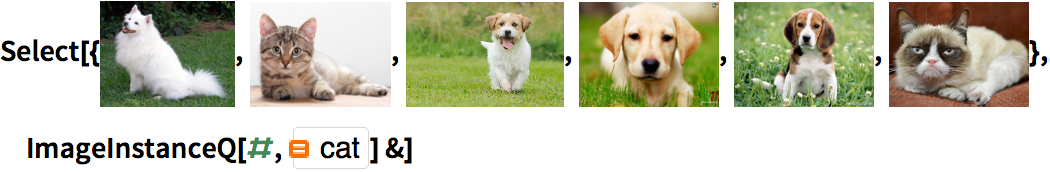Out=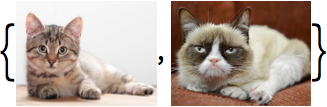Heres a geographic example of Select: find which cities in a list are less than 3000 miles from San Francisco.
Select cities whose distance from San Francisco is less than 3000 miles:
 In:=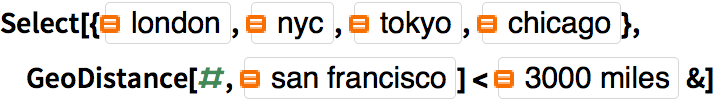Out=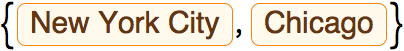ab test for equality ab test whether greater a≤b test whether less or equal a≥b test whether greater or equal If[test,u,v] give u if test is True and v if False Select[list,f] select elements that pass a test EvenQ[x] test whether even OddQ[x] test whether odd IntegerQ[x] test whether an integer PrimeQ[x] test whether a prime number LetterQ[string] test whether there are only letters MemberQ[list,x] test whether x is a member of list ImageInstanceQ[image,category] test whether image is an instance of category
28.1Test whether 123^321 is greater than 456^123. »
Expected output:
 Out[]=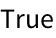Answer & check your solution
28.2Get a list of numbers up to 100 whose digits add up to less than 5. »
Expected output:
 Out[]=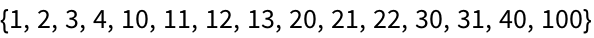Answer & check your solution
28.3Make a list of the first 20 integers, with prime numbers styled red. »
Expected output:
 Out[]=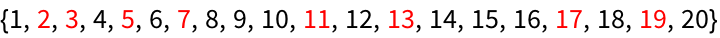Answer & check your solution
28.4Find words in WordList[ ] that both begin and end with the letter p»
Sample expected output:
 Out[]=Answer & check your solution
28.5Make a list of the first 100 primes, keeping only ones whose last digit is less than 3. »
Expected output:
 Out[]=Answer & check your solution
28.6Find Roman numerals up to 100 that do not contain I»
Expected output:
 Out[]=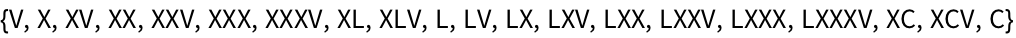Answer & check your solution
Expected output:
 Out[]=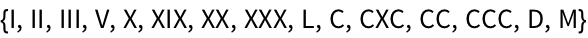Answer & check your solution
Expected output:
 Out[]=Answer & check your solution
28.9Get a list of words longer than 15 characters from the Wikipedia article on words. »
Sample expected output:
 Out[]=Answer & check your solution
28.10Starting from 1000, divide by 2 if the number is even, and compute 3#+1& if the number is odd; do this repeatedly 200 times (Collatz problem). »
Expected output:
 Out[]=Answer & check your solution
28.11Make a word cloud of 5-letter words in the Wikipedia article on computers. »
Sample expected output:
 Out[]=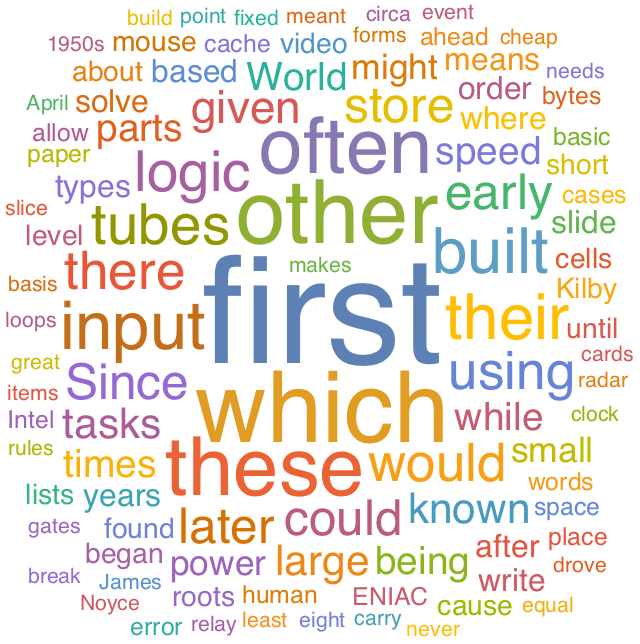Answer & check your solution
28.12Find words in WordList[ ] whose first 3 letters are the same as their last 3 read backward, but where the whole string is not a palindrome. »
Sample expected output:
 Out[]=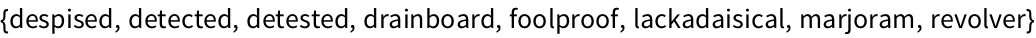Answer & check your solution
28.13Find all 10-letter words in WordList[ ] for which the total of LetterNumber values is 100. »
Sample expected output:
 Out[]=Answer & check your solution
+28.1Make a table of integers up to 25 where every integer ending in 3 is replaced with 0. »
Expected output:
 Out[]=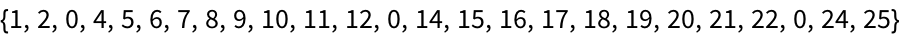Answer & check your solution
+28.2Use Table and If to make a 5×5 array that is 1 on its leading diagonal, and 0 otherwise.  »
Expected output:
 Out[]=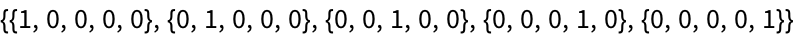Answer & check your solution
+28.3Get a list of numbers up to 1000 that are equal to 1 mod both 7 and 8. »
Expected output:
 Out[]=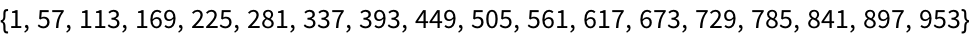Answer & check your solution
+28.4Make a list of numbers up to 100, where multiples of 3 are replaced by Black, multiples of 5 by White and multiples of 3 and 5 by Red»
Expected output:
 Out[]=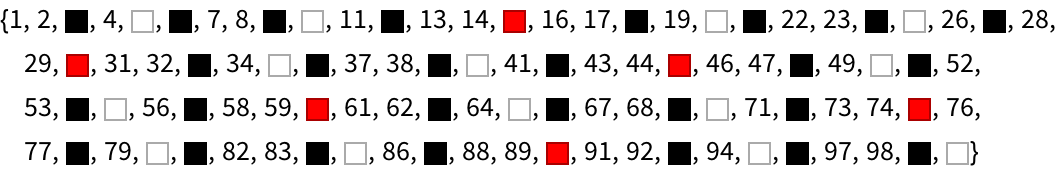Answer & check your solution
+28.5Use Select to get a list of planets whose mass is larger than Earth. »
Expected output:
 Out[]=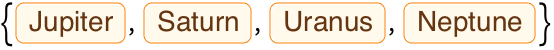Answer & check your solution
+28.6Make a 50×50 array plot in which a square at position i, j is black if Mod[i, j]==0»
Expected output:
 Out[]=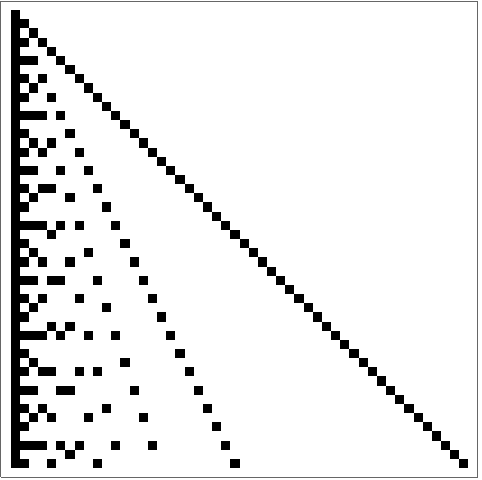Answer & check your solution
+28.7Make a 100×100 array plot in which a square is black if the values of both its x and y positions do not contain a 5. »
Expected output:
 Out[]=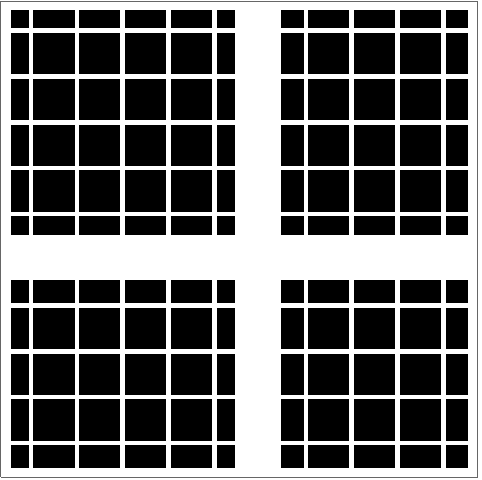Answer & check your solution
Because = means something else in the Wolfram Language. Youll get very strange results if you use = instead of == by mistake. (= is for assigning values of variables.) To avoid possible confusion, == is often read as double equals.
Why is and written as &&, not &?
Because & means other things in the Wolfram Language. For example its what ends a pure function.
How are ==, >, &&, etc. interpreted?
== is Equal, (!=) is Unequal, > is Greater, is GreaterEqual, < is Less, && is And, || is Or and ! is Not.
Theres an order of operations thats a direct analog of arithmetic. && is like ×, || is like +, and ! is like . So !p&&q means (not p) and q; !(p&&q) means not (p and q).
Yes. You can do things like Entity["Country", "Population"GreaterThan[]] to find implicit entities, then use EntityList to get explicit lists of entities.
• True and False are typically called Booleans in computer science, after George Boole from the mid-1800s. Expressions with &&, ||, etc. are often called Boolean expressions.
• In the Wolfram Language, True and False are symbols, and are not represented by 1 and 0 as in many other computer languages.
• If is often called a conditional. In If[test, then, else], the then and else arent computed unless the test says their condition is met.
• PalindromeQ directly tests if a string is a palindrome.
• In the Wolfram Language, x is a symbol (see Section 33) that could represent anything, so x==1 is just an equation, that isnt immediately True or False. x===1 (triple equals) tests whether x is the same as 1, and since it isnt, it gives False.